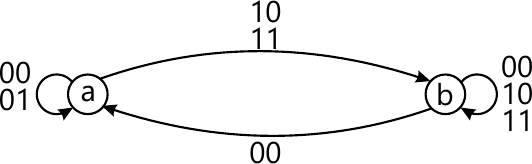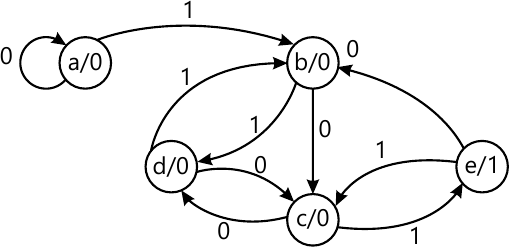MORE IN Design of Programming with Logic
VTU Computer Science (Semester 3)
Design of Programming with Logic
December 2011
Total marks: --
Total time: --
INSTRUCTIONS
(1) Assume appropriate data and state your reasons
(2) Marks are given to the right of every question
(3) Draw neat diagrams wherever necessary

1 (a) Draw the truth and explain how a TTL NAND gate works.
6 M
1 (b) An asymmetrical signal waveform is high for 2msec and low for 5msec. Find the frequency and the duty cycle L of the waveform.
4 M
1 (c) Discuss the positive and negative logic and list the equivalences in positive and negative logic.
5 M
1 (d) Draw the timing diagram and write a Verilog HDL code (using structural model) for the Boolean function Y=NAND (Y1, Y2); where Y1=A+B, Y2=B+C.
5 M

2 (a) A digital system is to be designed in which the months of the year is given as input in four bit form. The month January is represented as '0000', February as '0001' and so on. The output of the system should be '1' corresponding to the input of the month containing 31 days or otherwise it is '0'. Consider the excess numbers in the input beyond '1011' as don't care conditions. For this system of four variables (A, B, C, D), find the following:
(i) Boolean expression in ∑m and πM from
(ii) Write the truth table
(iii) Using K-map, simplify the Boolean expression of canonical min term form
(iv) Implement the simplified equation using NAND-NAND gates.
10 M
2 (b) Using Q-M method, simplify the expression f(A, B, C, D)=∑ (0,3,5,6,7,11,14). Write the gate diagram for the simplified equation using NAND-NAND gates.
10 M

3 (a) Implement the Boolean function expressed by POS, f(A, B, C, D)=π(1,2,5,6,9,12) using 8-to-1 MUX.
8 M
3 (b) Draw a PLA circuit to simultaneously realize the Boolean functions Y3=A'BC'; Y2=AC; Y1=AB'C+A'BC+ABC'; Y0=A'BC'+A'BC+A'B'C'+ABC.
6 M
3 (c) Implement a full adder using a 3-to-8 decoder.
6 M

4 (a) Draw the logic diagram of clocked 'D' flip-flop. Write its truth table, characteristics equation, state diagram and excitation table. Write is the drawback of SR flip-flop?
10 M
4 (b) Explain the Schmitt-Trigger transfer characteristic.
6 M
4 (c) Using behavioral model, write verilog HDL code a 'D' flip-flop.
4 M

5 (a) Using negative edge triggered JK flip-flop, draw the logic diagram of a 4-bit serial In-serial Out shift register. Draw the waveform to shift the binary number 1010 into this register. Also, draw the waveforms for four clock transitions when J=K=0 (Assuming the register has stored 1010 in it).
8 M
5 (b) How long will it take to shift the hexadecimal number 'AB' into the 54/74164 (SIPO) if 5MHz clock is connected to it? Also, mention the time required to extract an 8-bit number from the same register.
4 M
5 (c) With a neat diagram, explain a 4-bit universal shift register.
5 M
5 (d) Write Verilog code for switched-tail counter using 'assign' and 'always' statements.
3 M

6 (a) Mention any two differences between asynchronous counter. With a neat block diagram, output waveforms and truth table. Explain a 3-bit binary Ripple Down-Counter constructed using negative-edge trigged JK flip-flops.
10 M
6 (b) A 4-bit binary asynchronous counter is connected with a clock of 500 KHz frequency. Find the time period of the waveform at the output of the first and the last JK flip-flop.
2 M
6 (c) Design a synchronous mod-6 counter using JK flipflop.
8 M

7 (a) Compare Moore and Mealy model of synchronous sequential circuit.
6 M
7 (b) Design an asynchronous sequential logic circuit for state transition diagram shown in Fig.Q7(b).6 M
7 (c) Reduce state transition diagram (Moore model) of Fig.Q7(c) by,
(i) Row elimination method (ii) Implication table method.8 M

8 (a) Discuss the two drawbacks of resistive divider used in converting digital input to anlog output. Draw the schematic for a 4-bit binary ladder and explain how the digital to analog conversion is achieved using it.
10 M
8 (b) Using a schematic block diagram, briefly explain counter type ADC.
8 M
8 (c) A counter type 10 bit ADC is connected with MHz clock. Find :
(i) The average conversion time
(ii) The maximum conversion rate.
2 M

More question papers from Design of Programming with Logic